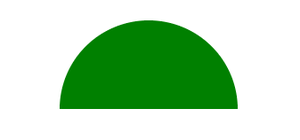# How to Draw a Semi-Circle using HTML and CSS ?

• Last Updated : 30 Jul, 2021

A Semi-Circle or a Half-Circle shape can be easily created using HTML and CSS. We will use the border-radius property to draw the desired output.

HTML Code: In this section we will create a simple “div” element using the <div> tag.

Attention reader! Don’t stop learning now. Get hold of all the important HTML concepts with the Web Design for Beginners | HTML  course.

## HTML

 ` ` `<``html` `lang``=``"en"``> ` ` `  `<``head``> ` `    ``<``meta` `charset``=``"UTF-8"``> ` `    ``<``meta` `name``=``"viewport"` `content``= ` `        ``"width=device-width, initial-scale=1.0"``> ` `    ``<``title``>Semi Circle ` ` ` ` `  `<``body``> ` `    ``<``div``> ` ` ` ` `  ` `

CSS Code: In this section we will first design the “div” box using simple CSS properties and then draw the semi-circle using the border-radius property.

## CSS

 ` `

Final Code: It is the combination of the above two code sections.

## HTML

 ` ` `<``html` `lang``=``"en"``> ` ` `  `<``head``> ` `    ``<``meta` `charset``=``"UTF-8"``> ` `    ``<``meta` `name``=``"viewport"` `content``= ` `        ``"width=device-width, initial-scale=1.0"``> ` ` `  `    ``<``title``>Semi Circle ` ` `  `    ``<``style``> ` `        ``* { ` `            ``margin: 0; ` `            ``padding: 0; ` `            ``background-color: white; ` ` `  `        ``} ` ` `  `        ``/* Using the border-radius property  ` `           ``to draw the semi-circle*/ ` `        ``div { ` `            ``position: absolute; ` `            ``top: 50%; ` `            ``left: 50%; ` `            ``transform: translate(-50%, -50%); ` `            ``height: 100px; ` `            ``width: 200px; ` `            ``border-radius: 150px 150px 0 0; ` `            ``background-color: green; ` `        ``} ` `    `` ` ` ` ` `  `<``body``> ` `    ``<``div``> ` ` ` ` `  ` `

Output:CSS is the foundation of webpages, is used for webpage development by styling websites and web apps.You can learn CSS from the ground up by following this CSS Tutorial and CSS Examples.

My Personal Notes arrow_drop_up
Recommended Articles
Page :